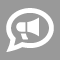#Blogs

## Math Equations Blogs

Hello Wzyant Academic Community and welcome to my blog section! This is where I am available for online chit-chat, educational assistance free of charge, business discussions & arrangements, and more! I am always eager to help and love to talk turkey with all realms of academia, so don't be shy and feel free to ask many questions!!!       P.S.  ∫∑∞√−±÷⁄∇¾φΩ

The general form for quadratic equations is:   Ax2+Bx+C   If A=1, you have x2+Bx+C=0, and the following procedure always works. If A ≠ 1, then Step 1 of both Scenarios below will always apply, but not Step 2.   The general form of the factors in this case will look like:   (x + D)*(x + E)   where D and E are factors of C whose sum is B.   In order to find D and E, its important to know whether they are positive or negative first. We find this out by using a simple procedure.   Scenario #1: Step 1: If C is negative: We know that D is positive and E is negative (or vice versa, it doesn't really matter which order you choose).   Step 2: If B is positive, we know that the factor of C with the larger absolute value will be the positive number. If B is negative, we know that the factor of C with the larger absolute value will be the negative... read more

Area, Volume and Circumference equations: Area of a Square A=S2 Area of a Triangle A=1/2bh Area of a Rectangle A=LW Right Triangle/Pythagorean Theorem a2+b2=c2 Area of Parallelogram A=bh Area of a Trapezoid A=1/2h(a+b) Area of a Circle A=πr2 Circumference of a Circle c=πd or c=2πr Volume of a Sphere V=4/3πr3 Surface Area of a Sphere SA=4πr2 Volume of a Cube V=s3 Volume of a Rectangular Solid V=lwh Slope of a line Equations Slope-intercept form y=mx+b m is the slope b is the y-intercept y is a y coordinate on the graph (that coincides with the line) x is an x coordinate on the graph (that coincides with the line) Horizontal line y=b Vertical line x=a Finding... read more

Awesome!  What an amazing way for elementary children to learn algebra!  Parents this will help you as well.  But...if you are still having problems, I do have a few Saturday slots available.   This has been a very busy few months!  For the most part I am totally booked.  The kids are doing great, grades are improving and self esteem is rising.   Practice, practice, practice is so important.  The more you do anything, the better you become.  Read, expand your mind, learn new words, repeat those times tables over and over until you don't even think about them anymore!   As we gear toward the upcoming Holidays, think about educational games and gifts.  The time you spend playing these games with your kids is priceless and fun!  Everyone wins!   The week of Thanksgiving, I will be taking a much needed vacation with my family, but I will be around during the Christmas break.  If you need... read more

I have noticed that the major problem students have while solving math problems is that they start working a problem out without pausing to wonder what the question is really asking. Read problem more than two times. Make sure you understand every key words. If not, go to the index of your math book and quickly look what those mathematical term means. After you find meanings of these special words, read the question again. Now, it is very possible that you have understood the problem. Don't start writing yet. Think how you might want to proceed. Now if you have an approach, start writing equations and try solving them. You can't solve an entire equation to find the answer in your head. So you must write each and every step clearly. You should double check each and every step.  Try this method to solve math problems from today. Good Luck.